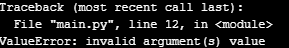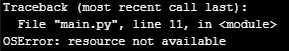# How to select ADC channel?

• When looking at the FiPy datasheet, it would seem that there is 2 different ADC channels, each having several corresponding pins.

I am having some problems understanding how to define which channel to use. From the documentation: https://docs.pycom.io/firmwareapi/pycom/machine/adc/

I am using following code to associate a pin with the ADC channel, but which one of the two channels?:

``````adc = ADC()
``````

From micropython documentation, it would seem that this could be selected this way:

``````adc = ADC()
apin = adc.channel(id = 2, pin = 'P16')
#or this way
apin = adc.channel(2, pin = 'P16')
``````

But both options produces following output:Now i have seen on the link below, that the ADC can be initated with code:

``````adc = ADC(0)
``````

which i would assume is ADC channel 1, but giving it (1) as an argument (channel 2 i assume) produces an error:So how do i select channel? I didn't find any useful post here, but thanks in advance.

EDIT: Just realized that P16 only is for ADC channel 1, so naturally it says resource not available, but trying to use channel 2 with, for instance, P9 also gives the resource not available error.

So i'm guessing that channel is simply decided by which pin you are using, and that you can only use one pin per channel at a time? Can someone confirm?

RE-EDIT: (sorry for all the edits, i'll try to test things out some more before i make posts in the future)

I tried this now:

``````adc=ADC()
``````

and got the resource not available error. So i'm still confused as to how this works? How do i activate the 2nd ADC channel?

Also i read somewhere that the ADC2 channel does not work when using WiFi, but that was an old post. Does that count for the FiPy in current versions?

/rm

• Still hoping for an answer. In short: How do i get to use ADC 2? How do i take readings from pin P3 for instance? Can i use both ADC channels, maybe even two pins at channel 1 and two pins at channel 2, at the same time?